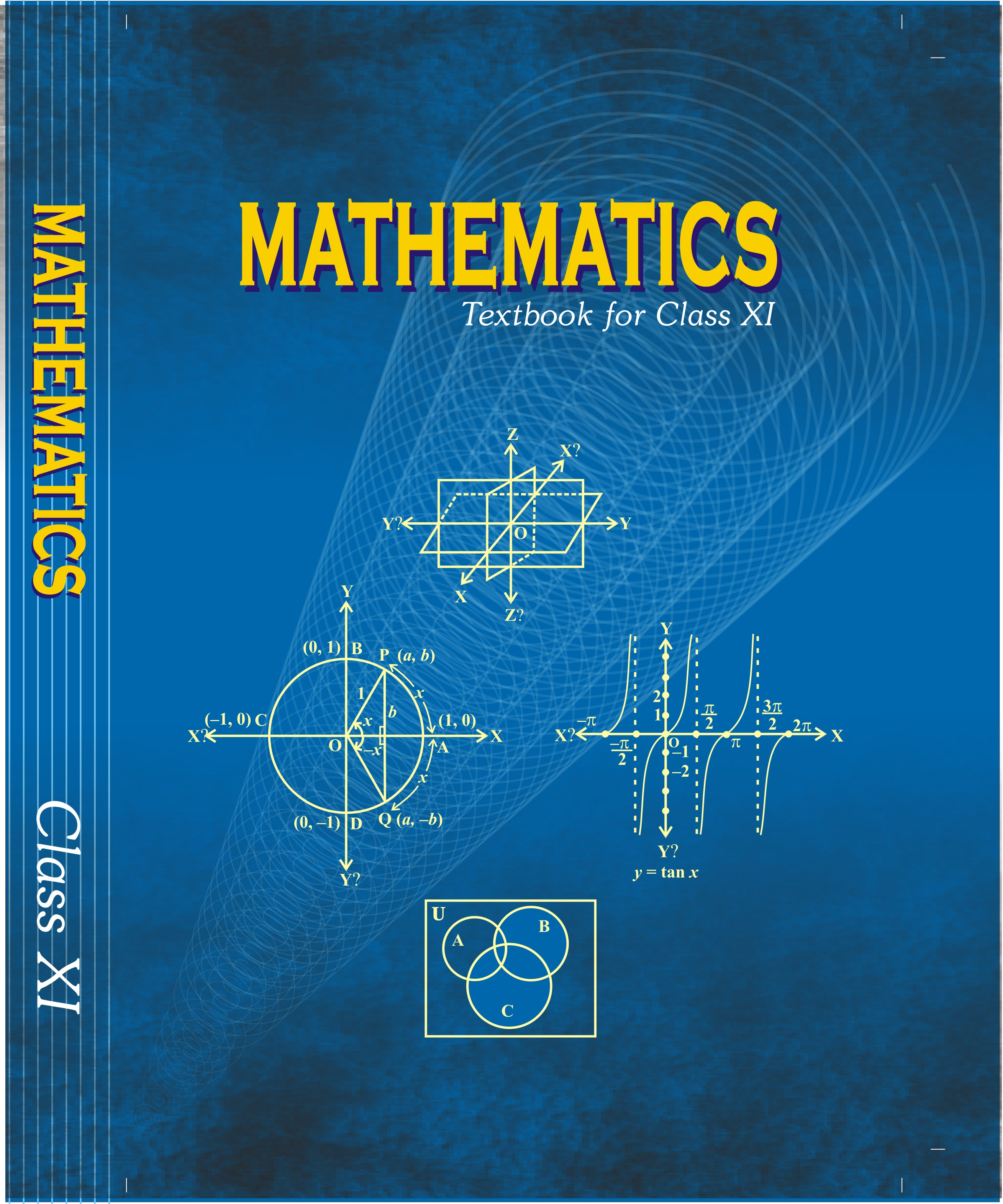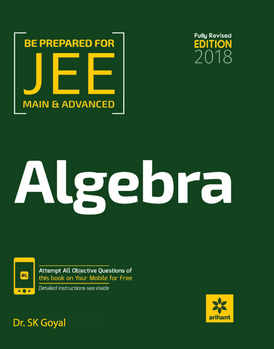# Matrices and Determinants (Weightage 7%)   Share

### Topics from Matrices and Determinants

• Test of consistency and solution of simultaneous linear equation in two or three variables using determinants and matrices (15 concepts)
• Properties of determinants, evaluation of determinants (14 concepts)
• Matrices, algebra of matrices, types of matrices (56 concepts)
• Adjoint and evaluation of inverse of a square matrix using deteminants and elementary transformations (14 concepts)
• Determinants and matrices of order two and three (2 concepts)

### Important Books for Matrices and Determinants

••Exams
Articles
Questions